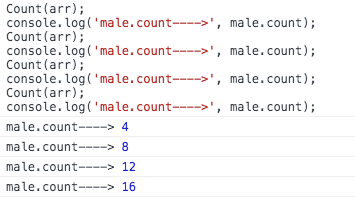JavaScript 函数式编程 实战开发

``const arr = [  {name: '赵信', gender: 1, age: 25, high: 176, weight: 62},   {name: '艾希', gender: 2, age: 23, high: 161, weight: 46},   {name: '阿狸', gender: 2, age: 27, high: 182, weight: 53},   {name: '盖伦', gender: 1, age: 27, high: 175, weight: 78},   {name: '沃里克', gender: 1, age: 42, high: 169, weight: 70},   {name: '安妮', gender: 2, age: 16, high: 153, weight: 43},   {name: '卡尔玛', gender: 2, age: 40, high: 168, weight: 48},   {name: '菲兹', gender: 0, age: 52, high: 163, weight: 50},   {name: '亚索', gender: 1, age: 35, high: 177, weight: 65},   {name: '锐雯', gender: 2, age: 33, high: 172, weight: 52}, ]``

``const male = {  count: 0,  list: [],};const MIN_AGE = 18;const Count = (arr) => {  for (const item of arr) {    if (      !item       || +item.age < +MIN_AGE       || `\${item.gender}` !== '1'    ) { continue }    male.count++;    male.list.push({      name: item.name,      high: item.high,    });  }}``

1. 不依赖外部状态，相同的输入永远得到相同的输出；

2. 没有副作用，不会修改入参或者全局变量。 // splice 说的就是你！``const Count = (arr, min) => {  // 创建一个局部变量  const res = {    count: 0,    list: [],  };  for (const item of arr) {    if (      !item       || +item.age < +min // 使用入参而不是全局变量      || `\${item.gender}` !== '1'    ) { continue }    res.count++;    res.list.push({      name: item.name,      high: item.high,    });  }  // 返回结果  return res;}``

``fun(a, b, c)  ->  fun(a)(b)(c)``

``fun(a, b, c) -> fun(a)(b, c) / fun(a, b)(c)``

``const sum = (x, y, z) => x + y + z;``

``const curry = (fn) => {    return function recursive(...args) {        // 如果args.length >= fn.length则表明传入了足够的参数，此时调用fn并返回        if (args.length >= fn.length) {            return fn(...args);        }        // 否则表明没有传入足够的参数，此时返回一个函数,用这个函数接受后面传递的新参数        return (...newArgs) => {            // 递归调用recursive函数,并返回            return recursive(...args.concat(newArgs));        };    };};``

``const Sum = curry(sum);      // -> [Function]Sum(10)(11)(12);             // -> 33const Sum10 = Sum(10);       // -> [Function]const Sum10_11 = Sum10(11);  // -> [Function]Sum10_11(12);                // -> 33``

``const compose = (f, g) => x => f(g(x))``

``const f = x => x + 1;const g = x => x * 2;const fg = compose(f, g);fg(1)  // ----> ?``

``filter18(arr);            // 从数组中返回年龄大于18岁的数据filterMale(arr);          // 从数组中筛选出男性数据并返回新数组pickNameHeight(arr);      // 获取数组中的姓名和身高字段并返回新数组log(arr);                 // 打印参数``

``log(pickNameHeight(filterMale(filter18(arr))));``

``const fun = compose(log, pickNameHeight, filterMale, filter18);fun(arr);``

1. 过滤出18岁以上的数据，首先需要实现一个用于比较大小的工具函数

`// 校验对象中的某个 key 是否大于临界值 val`
``function porpGt(key, val, item) {    return item[key] > val}``

``const cPropGt = curry(porpGt);        // porpGt(a, b, c) -> cPropGt(a)(b)(c)const filter18 = cPropGt('age')(18);  // cPropGt('age')(18)(item) -> filter18(item)arr.filter(filter18);                 // 返回 age 大于 18 的数据``

2. 过滤出男性，这需要一个判断等值的工具函数

``// 判断对象中的某个 key 是否等于临界值 valfunction porpEq(key, val, item) {  return `\${item[key]}` === `\${val}`}``

``const cPropEq = curry(porpEq);            // porpEq(a, b, c) -> cPropEq(a)(b)(c)const filterMale = cPropEq('gender')(1);  // cPropEq('gender')(1)(item) -> filterMale(item)arr.filter(filterMale);                   // 返回 gender 等于 1 的数据``

3. 记录身高和姓名，需要一个从对象中提取值的工具函数

``// 从对象中提取多个值并返回新的对象function pickAll(keys, item) {  const res = {};  keys.map(key => res[key] = item[key]);  return res;}``

``const cPickAll = curry(pickAll); const pickProps = cPickAll(['name', 'high']); arr.map(pickProps);   // 只保留 name 和 high``

``arr.filter(filter18)  .filter(filterMale)  .map(pickProps)``

``const Count = compose(  map(pickProps),  filter(filterMale),  filter(filter18),);Count(arr);``

## 关于作者白红宇是个全栈工程师，前端vue，小程序，app开发到后端框架设计，数据库设计，环境部署上线运维。